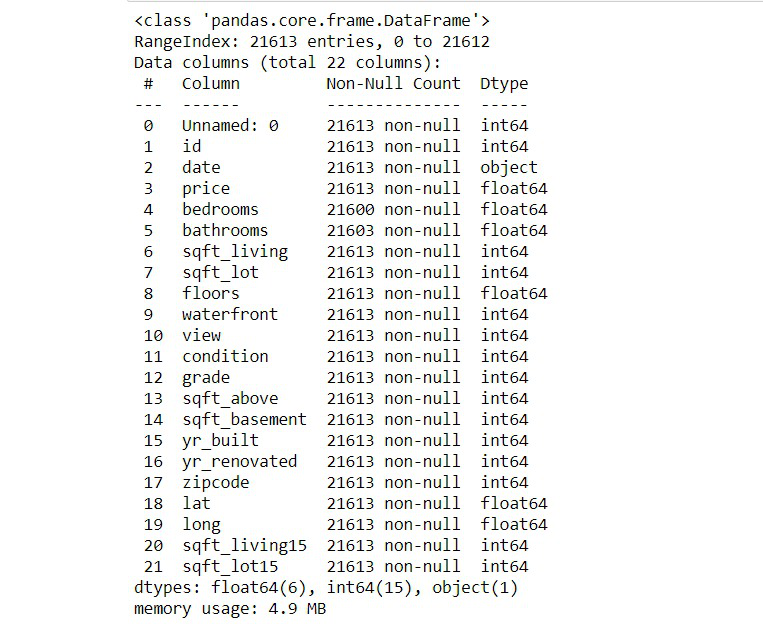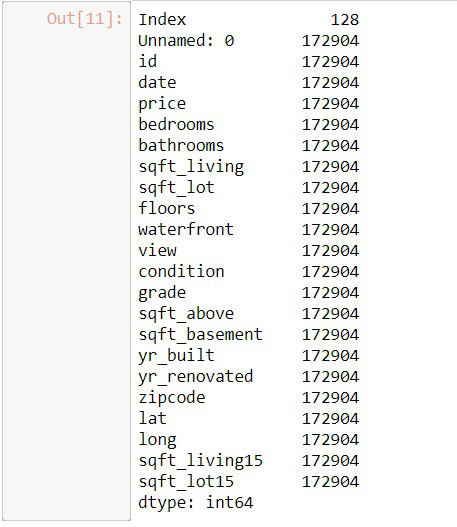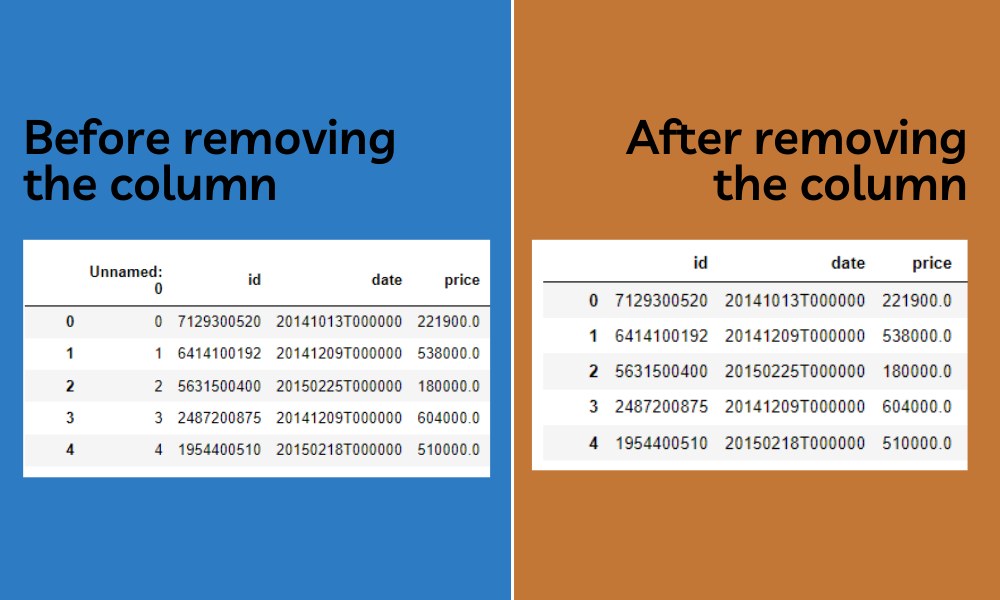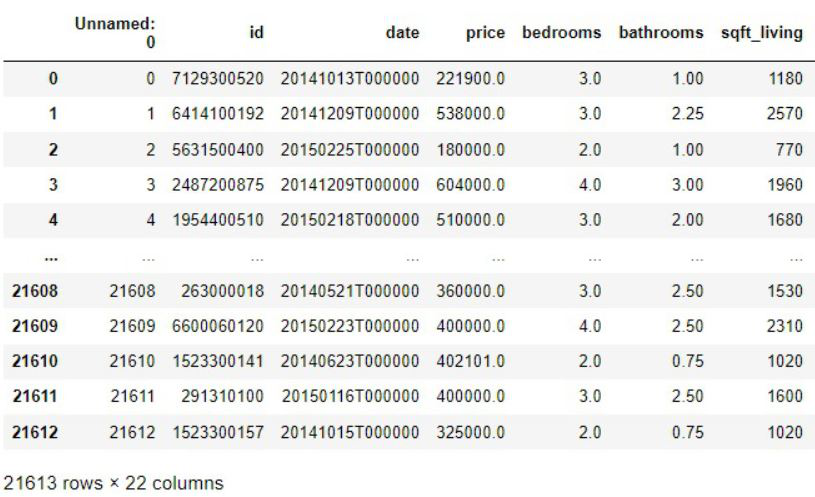GFG App
Open AppBrowser
Continue

When we work with pandas there is no doubt that you will always store the big data for better analysis. While dealing with the larger data, we should be more concerned about the memory that we use. There is no problem when you work with small datasets. It does not cause any issues. But we can program without dealing with memory issues in larger datasets.

Now we will see about how to reduce errors and memory consumption. It makes our work easier by speeding up the computation.

## Finding Memory usage

### Info() :

Info() methods return the summary of the dataframe.

syntax: DataFrame.info(verbose=None, buf=None, max_cols=None, memory_usage=None, show_counts=None, null_counts=None)

This will print the short and sweet summary of the dataframe. It will also give the memory usage taken by the data frame when we mention it as the parameter. For the parameter, we should mention memory_usage as “deep”.

## Python3

 `import` `pandas as pd`     `df ``=` `pd.read_csv(data.csv)` `df.info(memory_usage``=``"deep"``)`

Output:### Memory_usage():

Pandas memory_usage() function returns the memory usage of the Index. It returns the sum of the memory used by all the individual labels present in the Index.

Syntax: DataFrame.memory_usage(index=True, deep=False)

However, Info() only gives the overall memory used by the data. This function Returns the memory usage of each column in bytes. It can be a more efficient way to find which column uses more memory in the data frame.

## Python3

 `import` `pandas as pd`   `df ``=` `pd.read_csv(data.csv)` `df.memory_usage()`

Output:## Ways to optimize  memory in Pandas

### Changing numeric columns to smaller dtype:

This is a very simple method to preserve the memory used by the program. Pandas as default stores the integer values as int64 and float values as float64. This actually takes more memory. Instead, we can downcast the data types. Simply Convert the int64 values as int8 and float64 as float8. This will reduce memory usage. By converting the data types without any compromises we can directly cut the memory usage to near half.

Syntax:  columnName.astype(‘float16’)

Note: You cant store every value under int16 or float16. Some larger numbers still need to be stored as a larger datatype

Code:

## Python3

 `import` `pandas as pd` `df ``=` `pd.read_csv(``'data.csv'``)`   `# Downcasting float64 to float16` `df[``'price'``].memory_usage()`   `df[``'price'``] ``=` `df[``'price'``].astype(``'float16'``)` `df[``'price'``].memory_usage()`

Output:

```173032
43354```

It is common that we work with larger datasets, but there is no need of loading the entire dataset is necessary. Instead, we can load the specific columns on which you are going to work. By doing this we can restrict the amount of memory consumed to a very low value.

To do this, simply form a temporary dataset that contains only the values that you are going to work on.

## Python3

 `df.info(verbose ``=` `False``, memory_usage ``=` `'deep'``)` `df ``=` `df[[``'price'``, 'sqft_living]]` `df.info(verbose ``=` `False``, memory_usage ``=` `'deep'``)`

Output:

```<class 'pandas.core.frame.DataFrame'>
RangeIndex: 21613 entries, 0 to 21612
Columns: 22 entries, Unnamed: 0 to sqft_lot15
dtypes: float16(1), float64(5), int64(15), object(1)
memory usage: 4.8 MB```
```<class 'pandas.core.frame.DataFrame'>
RangeIndex: 21613 entries, 0 to 21612
Columns: 2 entries, price to sqft_living
dtypes: float16(1), int64(1)
memory usage: 211.2 KB```

In the above example, we can clearly see that loading the specific columns to the data frame reduces memory usage.

### Deleting

Deleting is one of the ways to save space. It might be the best solution, to any memory problem. We may unknowingly save many data frames for training and testing, which is further not used in the process. We can also delete the unused columns. By deleting these can save more space.  We can also delete the null columns present in the data frame which can also lead to saving more space.  We can use the del keyword followed by the item you want to delete. This should remove the item.

## Python3

 `# importing the modules` `import` `pandas as pd` `import` `numpy`   `# Reading the csv file` `df ``=` `pd.read_csv(``'data.csv'``)`   `# Deleting unwanted columns` `del` `df[``"Unnamed: 0"``]`### Changing categorical columns

When dealing with some datasets we might have some categorical columns in which the whole column only consists of a fixed set of values repeatedly. This type of data is called categorical values which are basically classifications or groups in which a large set of rows has a similar categorical value. While dealing with these types of data we can simply assign each categorical value to some alphabet or an integer[basically encoding]. This method can exponentially reduce the amount of memory used by the program.

syntax: df[‘column_name’].replace(‘largerValue’, ‘alphabet’, inplace=True)

## Python3

 `# importing the modules` `import` `pandas` `import` `numpy`   `# Reading the csv file` `df ``=` `pd.read_csv(``'data.csv'``)`   `# Memory usage before replacing` `df[``'bedrooms'``].memory_usage()` `# output--> 314640`   `# Replacing the categorical values` `df[``'bedrooms'``].replace(``'more than 2'``, ``1``, inplace``=``True``)` `df[``'bedrooms'``].replace(``'less than 2'``, ``0``, inplace``=``True``)`   `# Memory usage after replacing` `df[``'bedrooms'``].memory_usage()` `# output--> 173032`

Output:

```314640
173032```

After replacing the categorical values of the column bedrooms to 1’s and 0’s as you can see the memory usage has come to half.

### Importing data in chunks

While dealing with data it is obvious that in some situations that we need to work with big data that are generally in gigabytes. Your machine probably loads of computing power that is a memory to load all this data into the memory at once and it is nearly impossible. In this situation, pandas have a very handy method to load the data in chunks which will basically help us to iterate through the data set and load it in chunks instead of loading all the data at once and pushing your machine to its extreme.

To do this we need to pass the parameter called chunk size while reading the data. which will give us chunks of data that we need to concatenate into a complete data set. In this way, we can reduce the memory usage of the machine at once. and give some time to recover its computational power to work with the flow.

This method will return an iterable object of the dataset.

## Python3

 `# importing the mmodule` `import` `pandas`   `# Reading the data in chunks` `data ``=` `pandas.read_csv(``'data.csv'``, chunksize``=``1000``)`   `# Concatenating the chunks together` `df ``=` `pandas.concat(data)`

Output:My Personal Notes arrow_drop_up